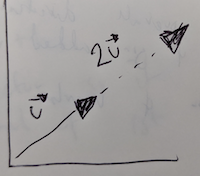## Vector ScalingVector scaling is the process of multiplying each value in the vector by some amount (hence "scalar").

$2 \begin{bmatrix} u_1 \\ u_2 \end{bmatrix} = \begin{bmatrix} 2 * u_1 \\ 2 * u_2 \end{bmatrix}$

The amount you scale the vector is often referred to as the scalar.

It can be visualised as growing or shrinking the arrow that represents the vector (see cover image).

In Perl, variables that contain a single value are called scalar variables.